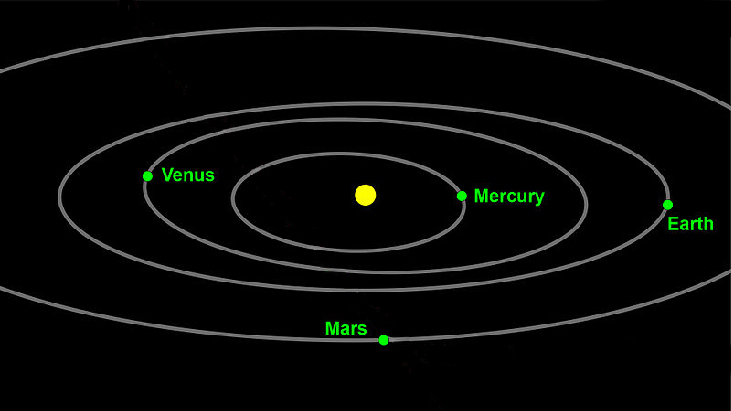# 10.4 Polar coordinates: graphs

 Page 1 / 16
In this section you will:
• Test polar equations for symmetry.
• Graph polar equations by plotting points.

The planets move through space in elliptical, periodic orbits about the sun, as shown in [link] . They are in constant motion, so fixing an exact position of any planet is valid only for a moment. In other words, we can fix only a planet’s instantaneous position. This is one application of polar coordinates    , represented as $\text{\hspace{0.17em}}\left(r,\theta \right).\text{\hspace{0.17em}}$ We interpret $\text{\hspace{0.17em}}r\text{\hspace{0.17em}}$ as the distance from the sun and $\text{\hspace{0.17em}}\theta \text{\hspace{0.17em}}$ as the planet’s angular bearing, or its direction from a fixed point on the sun. In this section, we will focus on the polar system and the graphs that are generated directly from polar coordinates.Planets follow elliptical paths as they orbit around the Sun. (credit: modification of work by NASA/JPL-Caltech)

## Testing polar equations for symmetry

Just as a rectangular equation such as $\text{\hspace{0.17em}}y={x}^{2}\text{\hspace{0.17em}}$ describes the relationship between $\text{\hspace{0.17em}}x\text{\hspace{0.17em}}$ and $\text{\hspace{0.17em}}y\text{\hspace{0.17em}}$ on a Cartesian grid, a polar equation describes a relationship between $\text{\hspace{0.17em}}r\text{\hspace{0.17em}}$ and $\text{\hspace{0.17em}}\theta \text{\hspace{0.17em}}$ on a polar grid. Recall that the coordinate pair $\text{\hspace{0.17em}}\left(r,\theta \right)\text{\hspace{0.17em}}$ indicates that we move counterclockwise from the polar axis (positive x -axis) by an angle of $\text{\hspace{0.17em}}\theta ,\text{\hspace{0.17em}}$ and extend a ray from the pole (origin) $\text{\hspace{0.17em}}r\text{\hspace{0.17em}}$ units in the direction of $\text{\hspace{0.17em}}\theta .\text{\hspace{0.17em}}$ All points that satisfy the polar equation are on the graph.

Symmetry is a property that helps us recognize and plot the graph of any equation. If an equation has a graph that is symmetric with respect to an axis, it means that if we folded the graph in half over that axis, the portion of the graph on one side would coincide with the portion on the other side. By performing three tests, we will see how to apply the properties of symmetry to polar equations. Further, we will use symmetry (in addition to plotting key points, zeros, and maximums of $\text{\hspace{0.17em}}r\right)\text{\hspace{0.17em}}$ to determine the graph of a polar equation.

In the first test, we consider symmetry with respect to the line $\text{\hspace{0.17em}}\theta =\frac{\pi }{2}\text{\hspace{0.17em}}$ ( y -axis). We replace $\text{\hspace{0.17em}}\left(r,\theta \right)\text{\hspace{0.17em}}$ with $\text{\hspace{0.17em}}\left(-r,-\theta \right)\text{\hspace{0.17em}}$ to determine if the new equation is equivalent to the original equation. For example, suppose we are given the equation $\text{\hspace{0.17em}}r=2\mathrm{sin}\text{\hspace{0.17em}}\theta ;$

This equation exhibits symmetry with respect to the line $\text{\hspace{0.17em}}\theta =\frac{\pi }{2}.$

In the second test, we consider symmetry with respect to the polar axis ( $\text{\hspace{0.17em}}x$ -axis). We replace $\text{\hspace{0.17em}}\left(r,\theta \right)\text{\hspace{0.17em}}$ with $\text{\hspace{0.17em}}\left(r,-\theta \right)\text{\hspace{0.17em}}$ or $\text{\hspace{0.17em}}\left(-r,\pi -\theta \right)\text{\hspace{0.17em}}$ to determine equivalency between the tested equation and the original. For example, suppose we are given the equation $\text{\hspace{0.17em}}r=1-2\mathrm{cos}\text{\hspace{0.17em}}\theta .$

The graph of this equation exhibits symmetry with respect to the polar axis.

In the third test, we consider symmetry with respect to the pole (origin). We replace $\text{\hspace{0.17em}}\left(r,\theta \right)\text{\hspace{0.17em}}$ with $\text{\hspace{0.17em}}\left(-r,\theta \right)\text{\hspace{0.17em}}$ to determine if the tested equation is equivalent to the original equation. For example, suppose we are given the equation $\text{\hspace{0.17em}}r=2\mathrm{sin}\left(3\theta \right).$

$\begin{array}{c}\text{\hspace{0.17em}}\text{\hspace{0.17em}}\text{\hspace{0.17em}}\text{\hspace{0.17em}}\text{\hspace{0.17em}}r=2\mathrm{sin}\left(3\theta \right)\\ -r=2\mathrm{sin}\left(3\theta \right)\end{array}$

The equation has failed the symmetry test , but that does not mean that it is not symmetric with respect to the pole. Passing one or more of the symmetry tests verifies that symmetry will be exhibited in a graph. However, failing the symmetry tests does not necessarily indicate that a graph will not be symmetric about the line $\text{\hspace{0.17em}}\theta =\frac{\pi }{2},\text{\hspace{0.17em}}$ the polar axis, or the pole. In these instances, we can confirm that symmetry exists by plotting reflecting points across the apparent axis of symmetry or the pole. Testing for symmetry is a technique that simplifies the graphing of polar equations, but its application is not perfect.

#### Questions & Answers

A laser rangefinder is locked on a comet approaching Earth. The distance g(x), in kilometers, of the comet after x days, for x in the interval 0 to 30 days, is given by g(x)=250,000csc(π30x). Graph g(x) on the interval [0, 35]. Evaluate g(5)  and interpret the information. What is the minimum distance between the comet and Earth? When does this occur? To which constant in the equation does this correspond? Find and discuss the meaning of any vertical asymptotes.
Kaitlyn Reply
The sequence is {1,-1,1-1.....} has
amit Reply
circular region of radious
Kainat Reply
how can we solve this problem
Joel Reply
Sin(A+B) = sinBcosA+cosBsinA
Eseka Reply
Prove it
Eseka
Please prove it
Eseka
hi
Joel
June needs 45 gallons of punch. 2 different coolers. Bigger cooler is 5 times as large as smaller cooler. How many gallons in each cooler?
Arleathia Reply
7.5 and 37.5
Nando
find the sum of 28th term of the AP 3+10+17+---------
Prince Reply
I think you should say "28 terms" instead of "28th term"
Vedant
the 28th term is 175
Nando
192
Kenneth
if sequence sn is a such that sn>0 for all n and lim sn=0than prove that lim (s1 s2............ sn) ke hole power n =n
SANDESH Reply
write down the polynomial function with root 1/3,2,-3 with solution
Gift Reply
if A and B are subspaces of V prove that (A+B)/B=A/(A-B)
Pream Reply
write down the value of each of the following in surd form a)cos(-65°) b)sin(-180°)c)tan(225°)d)tan(135°)
Oroke Reply
Prove that (sinA/1-cosA - 1-cosA/sinA) (cosA/1-sinA - 1-sinA/cosA) = 4
kiruba Reply
what is the answer to dividing negative index
Morosi Reply
In a triangle ABC prove that. (b+c)cosA+(c+a)cosB+(a+b)cisC=a+b+c.
Shivam Reply
give me the waec 2019 questions
Aaron Reply

### Read also:

#### Get the best Algebra and trigonometry course in your pocket!

Source:  OpenStax, Algebra and trigonometry. OpenStax CNX. Nov 14, 2016 Download for free at https://legacy.cnx.org/content/col11758/1.6
Google Play and the Google Play logo are trademarks of Google Inc.

Notification Switch

Would you like to follow the 'Algebra and trigonometry' conversation and receive update notifications?ByByBy RhodesBy